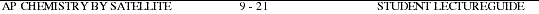###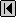Go to Main Index

AP Chemistry by Satellite Lectureguide
Student Edition
Molecular Geometry
Chapter 9

Objectives

Following your study of this chapter, you should be able to

1. define the terms bond angle and bond length in the molecules of covalent compounds.
2. distinguish between bonding pairs and nonbonding pairs of electrons in the molecules of covalent compound.
3. differentiate between the electron-pair geometry and the molecular geometry for any simple covalent molecule.
4. apply the molecular geometry using valence-shell electron pair repulsion (VSEPR) model to predict molecular geometry.
5. describe molecular geometries that result from bond angles in a given covalent molecule.
6. describe the effect of nonbonding electrons on bond angle in a given series of covalent molecules.
7. identify axial and equatorial atoms in simple covalent molecules.
8. identify the molecular geometry about any non-terminal atom in any molecule lacking a single central atom.
9. define the term polar and nonpolar as it is used in covalent molecules.
10. define the term dipole moment and predict its presence or absence in a covalent molecule.
11. define the term overlap and diagram the orbital overlap of two s orbitals, of an s and a p orbital, and of two p orbitals.
12. distinguish between sigma and a pi chemical bonds.
13. describe how a set of hybrid orbitals differ from a collection of atomic orbitals.
14. show how the geometry of a covalent molecule to a particular set of hybrid orbitals on a central atom.
15. identify the atomic orbitals used to bind atoms in covalent molecules containing multiple bonds.
16. explain the meaning of the concept of delocalized bonding electrons.
17. list characteristic physical properties of the elemental form of oxygen, sulfur, carbon and silicon.
18. draw and identify the molecular geometry of the elemental form of oxygen, sulfur, carbon and silicon.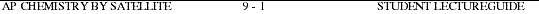1a. Sketch a five-atom molecule that has a central atom to which the remaining four atoms are independently attached. All bond angles are equal and all bond lengths are equal. In your sketch label bond angels and bond lengths.2a. Draw the Lewis electron dot structure for CCl4 and identify all the bonding pairs and nonbonding pairs of electrons.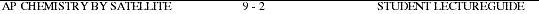3. Differentiate between electron-pair geometry and molecular geometry.4. List the steps used to predict the molecular geometry using VSEPR.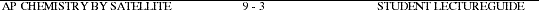5. Complete the following table. Note: Be sure to include all possible combinations of bonding and nonbonding electrons on the central atom.)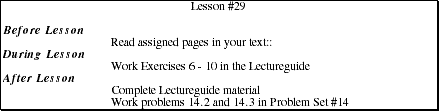6. How does the change in the number of bonding electrons and nonbonding electrons affect the molecular geometry for the series AB4, AB3N, AB2N2. (Note: A is the symbol for the central atom, B is the symbol for a bonding group of electrons and N is the symbol for a nonbonding pair of electrons.)7. In which molecular geometry is it necessary to differentiate between axial and equatorial atoms?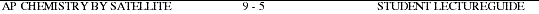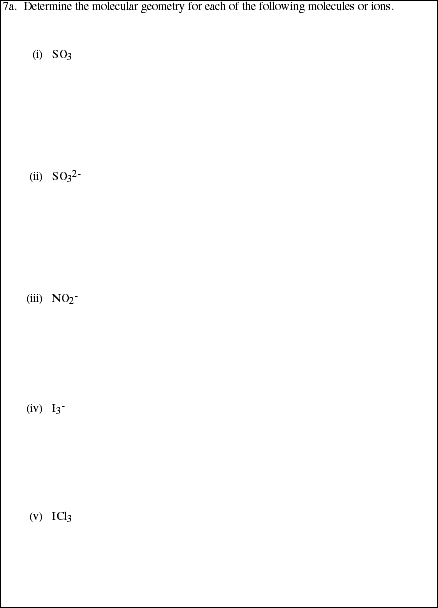8. How does predicting molecular geometries differ for molecules with multiple 'central atoms' compared to molecules with a single central atom?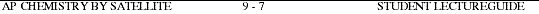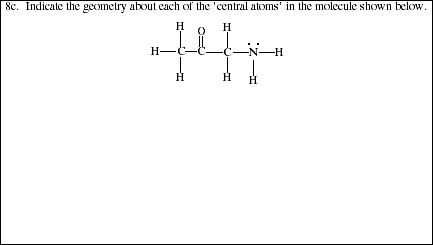9a. Based on a Lewis structure, explain how using the terminal atoms and lone pairs of electrons on the central atom one can distinguish between polar molecules and a nonpolar molecules.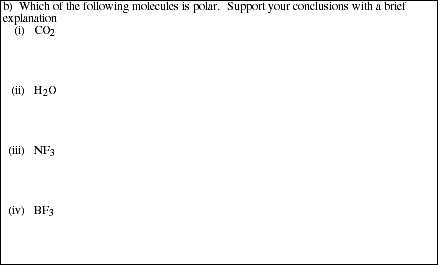10a. Define the term dipole moment.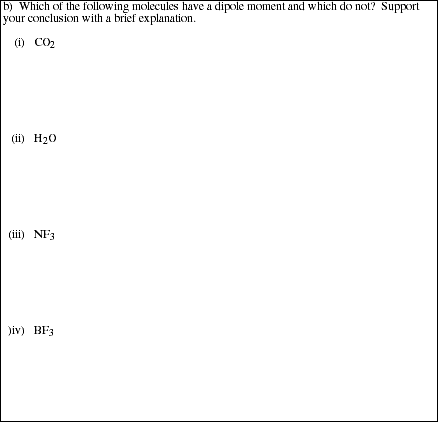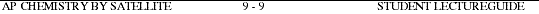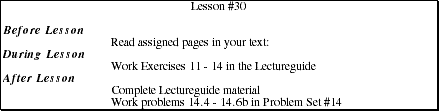11a. Define the term overlap as it relates to interaction of atomic orbitals on atoms.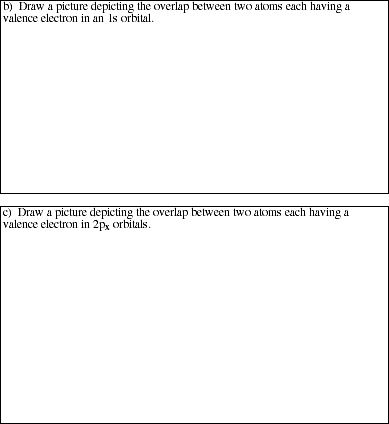12. Explain the difference between the model for a sigma (s) bond and for a pi (p) bond. Clarify the distinction between the overlaps by drawing a picture of two 'p' orbitals forming a sigma (s) bond and two 'p' orbitals forming a pi (p) bond.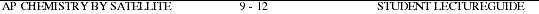13. Given four atomic orbitals, an s orbital and three p orbitals and the resulting set of four sp3 hybrid orbitals, explain the difference in shape, orientation, size and14. Complete the following table by filling in the missing information.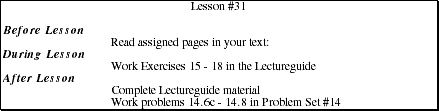15. Which hybrid orbitals are used to describe multiple bonding between carbon and oxygen in the following molecules?16. Explaining the meaning of the term delocalized as it applies to binding electrons and how the term is used to explain the structure of benzene, C6H6.17. Describe some of the physical properties (phase, color, melting point, boiling point) of oxygen, ozone, sulfur, carbon and silicon.18. Draw the Lewis structure of each of the elemental forms of oxygen, ozone, sulfur, carbon (simple sketch of graphite or diamond) and silicon.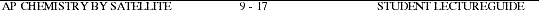Problem Set #14
AP Chemistry by Satellite

ALL work must be shown in all problems for full credit.

PS14.1. Complete the following table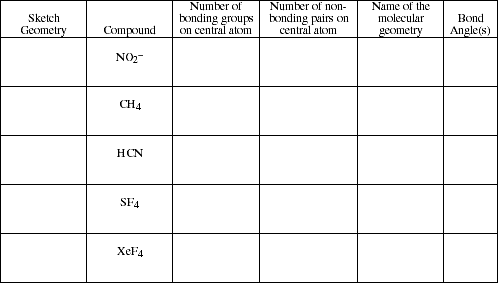PS14.2. Use simple structure and bonding models to account for each of the following.a). The H-N-H bond angle is 107.5 º in NH3.b). The I3- ion is linear.PS14.3. Which of the molecules listed in Problem #14.1 above are polar and which are nonpolar?PS14.4. Consider theLewis structure for glycine, the simplest amino acid:a). What are the approximate bond angles about each of the two carbon atoms, and what are the hybredizations of the orbitals on each of them?b). What are the hybridizations of the orbitals on the two oxygens and the nitrogen atom, and what are the approximate bond angles about the nitrogen?c). What is the total number of s bonds in the entire molecule? Sigma (s) bonds?PS14.5. Indicate the hybridzation on the central atom for each of molecules in Problem 14.1 above.PS14.6. Indicate the atomic orbitals on each atom in the following molecules which are involved in forming the covalent bond.

a). H-Fb). F2c).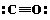PS14.7a. Draw two resonance structures for the nitrite ion, NO2-.b). What is the hybridization around the nitrogen atom in this polyatomic ion?c). Describe the pi-bonding in the polyatomic ion.d). Label both the sigma and the pi bonds as either localized or delocalized.PS14.8. Use simple structure and bonding models to account for the following. The bond lengths in CO32- are all identical and are shorter than a carbon- oxygen single bond.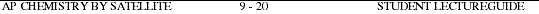Microcomputer software

Project SERAPHIM
Dr. John Moore
Department of Chemistry
University of Wisconsin

AP401 ISOMERS
VSEPRComputer Aided Instruction for General Chemistry by William Butler & Raymond Hough Drill-and-practice software
\$40 (4-disk set)

John Wiley & Sons, Inc.
605 3rd Avenue
New York, NY 10158
(this software may not be available)

Diskette #2 Molecular Bonding and Structure